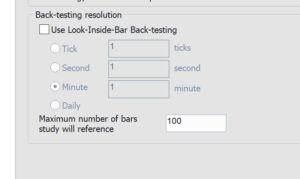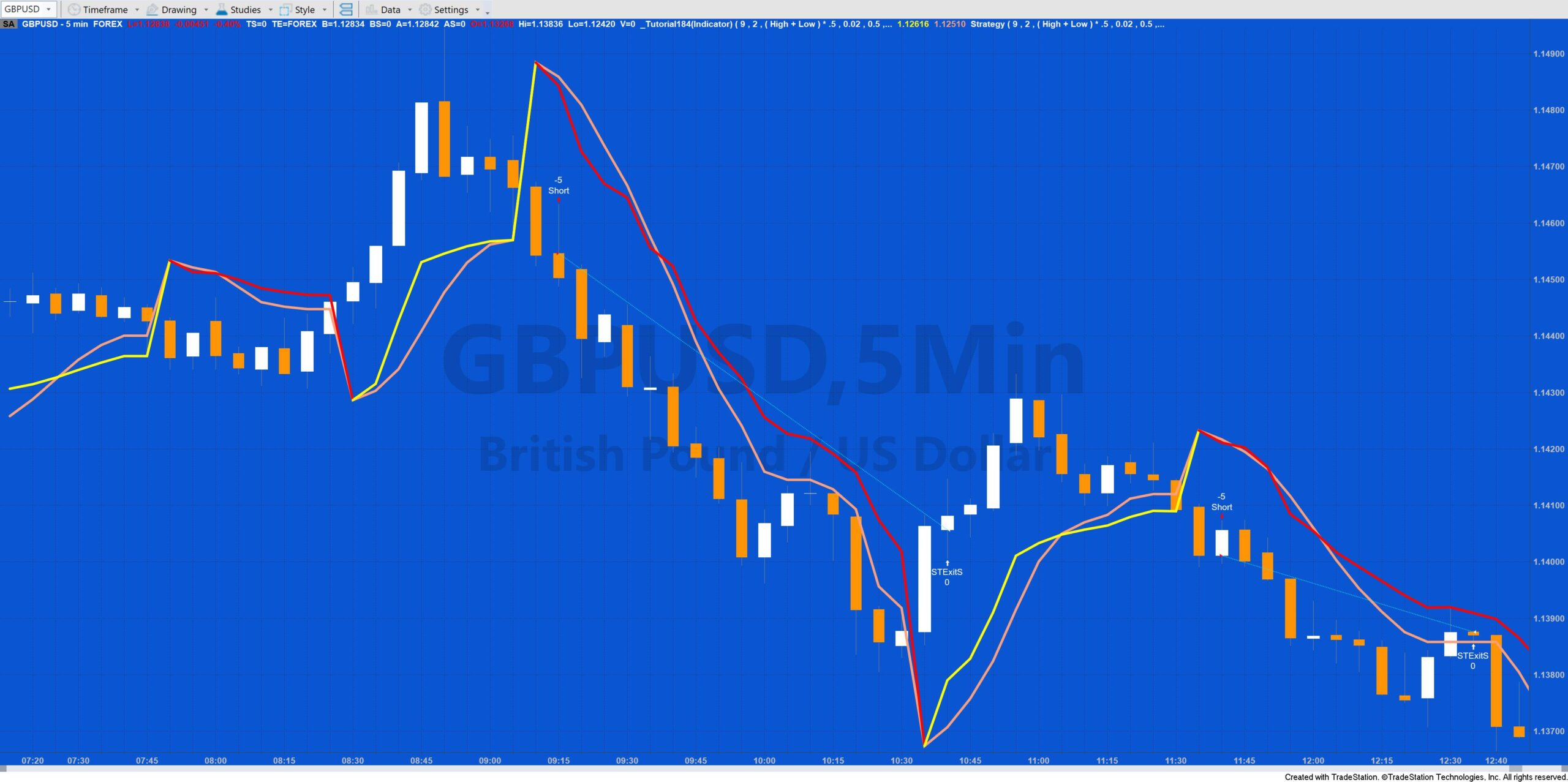# Tutorial 184 | SuperTrend strategy framework and indicator

Tutorial 184 is based on the Markplex SuperTrend indicator which is a stop and reverse (SAR) indicator that trails price action See the original indicator: tutorial 173 here.

This implementation calculates the Average True Range (ATR) of the average price using the exponential moving average. A user input multiple of the ATR is added to or subtracted from the average price to give Upper and Lwr respectively, depending on the direction. Direction is determined by the Close of a bar relative to the value of the SuperTrend, Upper and Lwr using the following code:

`If C > SupTrend and C > Lwr then Dir = 1else if C < SupTrend and C < Upper then Dir = -1;`

When the direction is up (Dir = 1), the SupTrend value can only increase. When the direction is down, the SupTrend can only decrease.

There are many different interpretations of the so-called SuperTrend indicator. In this Markplex version the SuperTrend value is also tightened (i.e. moved closer to price) by using an acceleration factor which increases incrementally each bar by user input: AFStep up to a a maximum value of user input: MaxAF.

In addition a ‘Supertrend’ exit is calculated (SupTrendExit). The value of this is set to the value of SupTrend when direction changes and is then calculated using:

`SupTrendExit = MaxList( SupTrendExit, L - ( ( L - SupTrendExit ) * ( 1 - AFExit ) ) )`

when Dir = 1, otherwise by:

`SupTrendExit = MinList( SupTrendExit, H + ( SupTrendExit - H ) * ( 1 - AFExit ) )`

A buy market order is generated when Dir changes from -1 to 1, provided that LongFilter1, LongFilter2 and LongFilter3 all evaluate to true and the program is not currently in a trade.

A sell short market order is generated when Dir changes from 1 to -1, provide that ShortFilter1, ShortFilter2 and ShortFilter3 all evaluate to true and the program is not currently in a trade.

When in a long position and the close crosses under SupTrendExit a market order to exit the trade next bar is generated provided the trade has been in the trade more than the user input: MinBarsNTrade number of bars. Similarly, when in a short position and the close goes above SupTrendExit a market order to exit the trade next bar is generated provided the trade has been in the trade more than the user input: MinBarsNTrade number of bars. The user could experiment with replacing this functionality will other types of stop, for example a trailing stop.The tutorial gives an example of LongFilter1 and ShortFilter1 using an implementation of the Moving Average Gap Ratio. If this is used, the user will need to change the strategies’ “Maximum Number of Bars Study will reference” to be equal to the value of SlowLength. The user can experiment with replacing this filter with their own as the strategy tends to get ‘whipsawed’ in non-trending markets.

To use this strategy make sure that “Enable intrabar order generation and calculation” is not selected.

### Tutorial 184 (indicator and strategy) applied to a chart